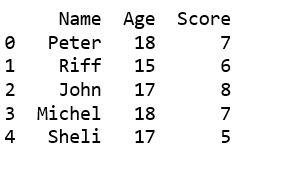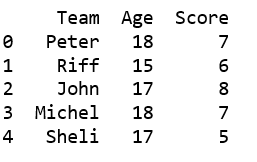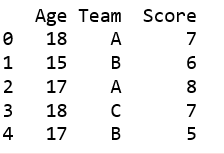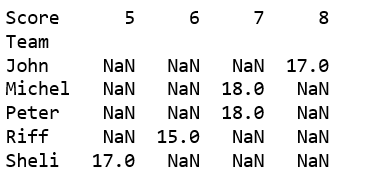# Creating a Pandas dataframe using list of tuples

We can create a DataFrame from a list of simple tuples, and can even choose the specific elements of the tuples we want to use.

Code #1: Simply passing tuple to DataFrame constructor.

 `# import pandas to use pandas DataFrame ` `import` `pandas as pd ` ` `  `# data in the form of list of tuples ` `data ``=` `[(``'Peter'``, ``18``, ``7``), ` `        ``(``'Riff'``, ``15``, ``6``), ` `        ``(``'John'``, ``17``, ``8``), ` `        ``(``'Michel'``, ``18``, ``7``), ` `        ``(``'Sheli'``, ``17``, ``5``) ] ` ` `  ` `  `# create DataFrame using data ` `df ``=` `pd.DataFrame(data, columns ``=``[``'Name'``, ``'Age'``, ``'Score'``]) ` ` `  `print``(df)  `

Output:Code #2: Using `from_records()`

 `import` `pandas as pd ` ` `  `# data in the form of list of tuples ` `data ``=` `[(``'Peter'``, ``18``, ``7``), ` `        ``(``'Riff'``, ``15``, ``6``), ` `        ``(``'John'``, ``17``, ``8``), ` `        ``(``'Michel'``, ``18``, ``7``), ` `        ``(``'Sheli'``, ``17``, ``5``) ] ` ` `  ` `  `# create DataFrame using data ` `df ``=` `pd.DataFrame.from_records(data, columns ``=``[``'Team'``, ``'Age'``, ``'Score'``]) ` ` `  `print``(df)  `

Output:Code #3: Using `from_items()`

 `import` `pandas as pd ` ` `  `# data in the form of list of tuples ` `data ``=` `[ ` `(``'Age'``, [``18``, ``15``, ``17``, ``18``, ``17``]), ` `(``'Team'``, [``'A'``, ``'B'``, ``'A'``, ``'C'``, ``'B'``]), ` `(``'Score'``, [``7``, ``6``, ``8``, ``7``, ``5``]), ` `] ` ` `  `# create DataFrame using data ` `df ``=` `pd.DataFrame.from_items(data) ` ` `  `print``(df)  `

Output:Code #4: For pivoting it possible.

 `# import pandas to use pandas DataFrame ` `import` `pandas as pd ` ` `  `# data in the form of list of tuples ` `data ``=` `[(``'Peter'``, ``18``, ``7``), ` `        ``(``'Riff'``, ``15``, ``6``), ` `    ``(``'John'``, ``17``, ``8``), ` `    ``(``'Michel'``, ``18``, ``7``), ` `    ``(``'Sheli'``, ``17``, ``5``) ] ` ` `  ` `  `# create DataFrame using data ` `df ``=` `pd.DataFrame(data, columns ``=``[``'Team'``, ``'Age'``, ``'Score'``]) ` ` `  `a ``=` `df.pivot(``'Team'``, ``'Score'``,``'Age'``) ` `print``(a) `

Output:My Personal Notes arrow_drop_upCheck out this Author's contributed articles.

If you like GeeksforGeeks and would like to contribute, you can also write an article using contribute.geeksforgeeks.org or mail your article to contribute@geeksforgeeks.org. See your article appearing on the GeeksforGeeks main page and help other Geeks.

Please Improve this article if you find anything incorrect by clicking on the "Improve Article" button below.

Article Tags :

Be the First to upvote.

Please write to us at contribute@geeksforgeeks.org to report any issue with the above content.